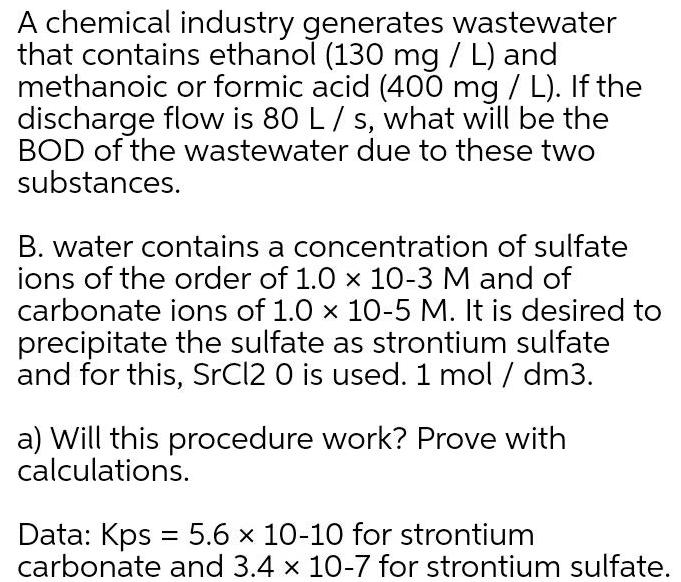Physical Chemistry
Equilibrium
A chemical industry generates wastewater that contains ethanol 130 mg L and methanoic or formic acid 400 mg L If the discharge flow is 80 L s what will be the BOD of the wastewater due to these two substances B water contains a concentration of sulfate ions of the order of 1 0 x 10 3 M and of carbonate ions of 1 0 x 10 5 M It is desired to precipitate the sulfate as strontium sulfate and for this SrCl2 0 is used 1 mol dm3 a Will this procedure work Prove with calculations Data Kps 5 6 x 10 10 for strontium carbonate and 3 4 x 10 7 for strontium sulfate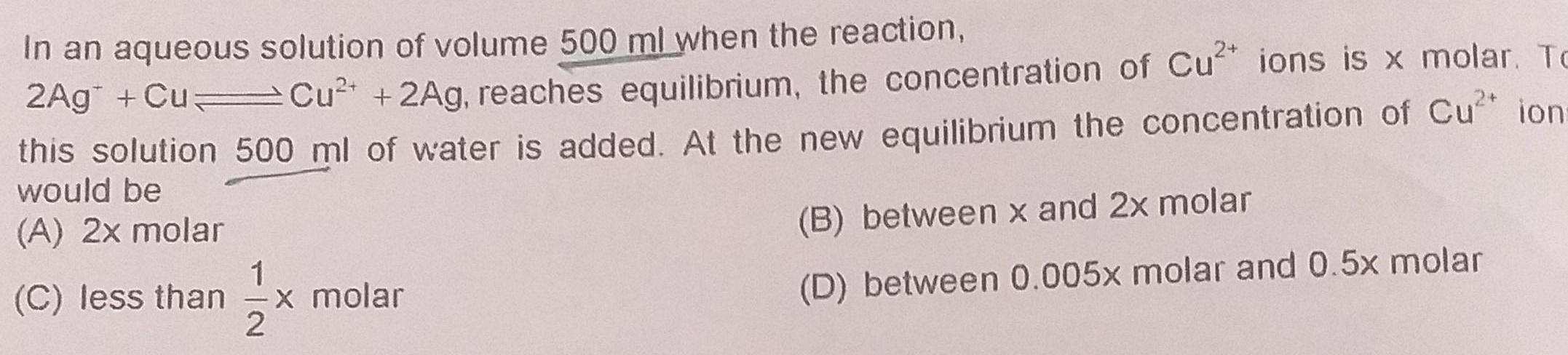Physical Chemistry
Equilibrium
In an aqueous solution of volume 500 ml when the reaction 2Ag Cu Cu 2Ag reaches equilibrium the concentration of Cu ions is x molar To this solution 500 ml of water is added At the new equilibrium the concentration of Cu ion would be A 2x molar 1 C less than x molar 2 B between x and 2x molar D between 0 005x molar and 0 5x molar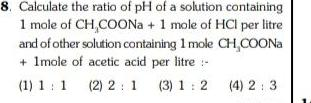Physical Chemistry
Equilibrium
8 Calculate the ratio of pH of a solution containing 1 mole of CH COONa 1 mole of HCI per litre and of other solution containing 1 mole CH COONa 1mole of acetic acid per litre 1 1 1 2 2 1 3 1 2 4 2 3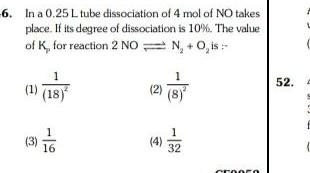Physical Chemistry
Equilibrium
6 In a 0 25 L tube dissociation of 4 mol of NO takes place If its degree of dissociation is 10 The value of K for reaction 2 NO N O is 1 18 3 7 6 16 4 8 1 32 CROOCO 52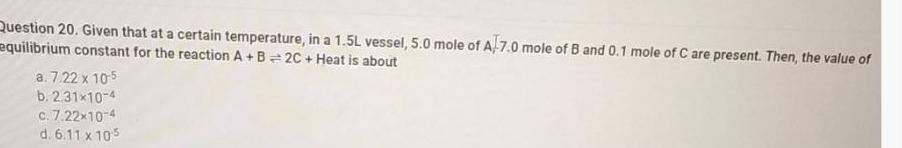Physical Chemistry
Equilibrium
Question 20 Given that at a certain temperature in a 1 5L vessel 5 0 mole of A 7 0 mole of B and 0 1 mole of C are present Then the value of equilibrium constant for the reaction A B 2C Heat is about a 7 22 x 10 5 b 2 31 10 4 c 7 22 10 4 d 6 11 x 105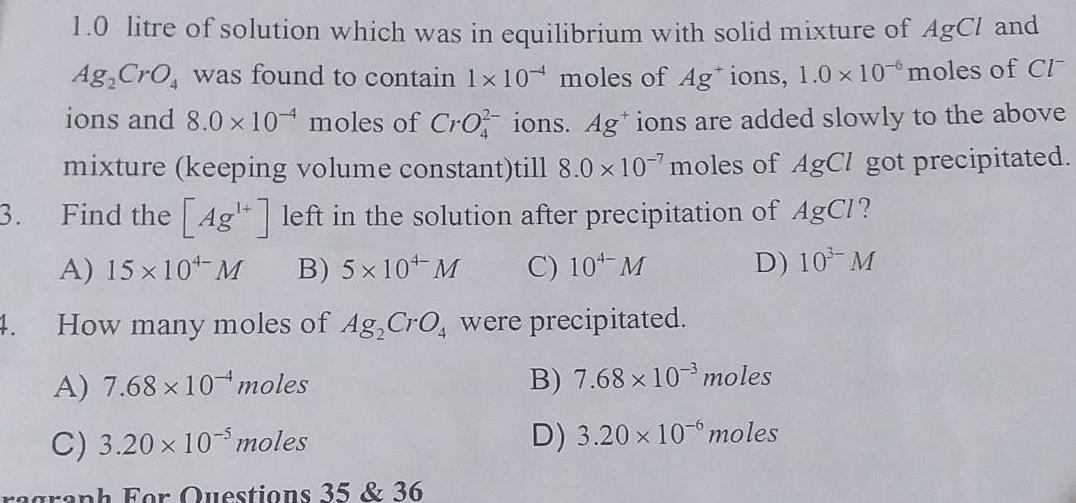Physical Chemistry
Equilibrium
1 0 litre of solution which was in equilibrium with solid mixture of AgCl and Ag CrO was found to contain 1x 10 moles of Ag ions 1 0 x 10 moles of Cl ions and 8 0 x 10 moles of Cro ions Ag ions are added slowly to the above mixture keeping volume constant till 8 0 x 10 7 moles of AgCl got precipitated 3 Find the 4g left in the solution after precipitation of AgCl A 15 10 M B 5 10 M C 10 M D 10 M 4 How many moles of Ag CrO were precipitated A 7 68 x 10 moles C 3 20 x 10 moles ragraph For Questions 35 36 B 7 68 x 10 moles D 3 20 x 10 moles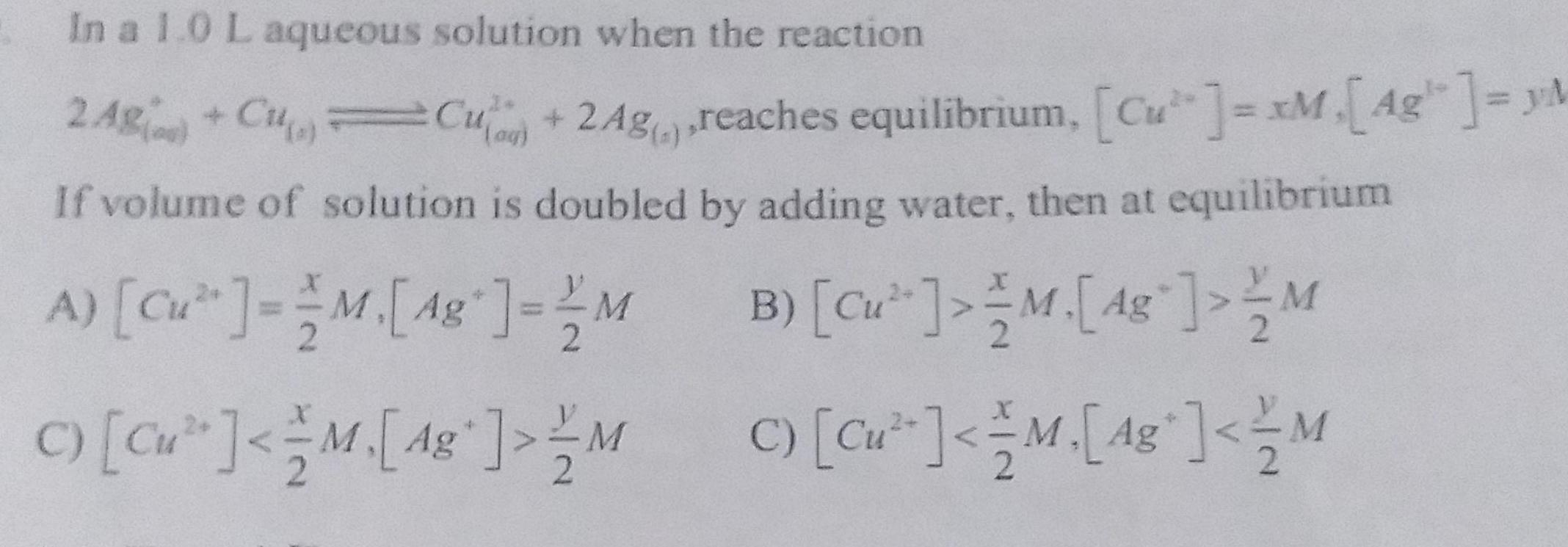Physical Chemistry
Equilibrium
In a 1 0 L aqueous solution when the reaction 2 AB g Cu a Cu 2Ag reaches equilibrium Cu xM A4g M If volume of solution is doubled by adding water then at equilibrium A Cu M 48 MB Cu M 48 M 2 C Cu M 48 MC Cu M 48 M 2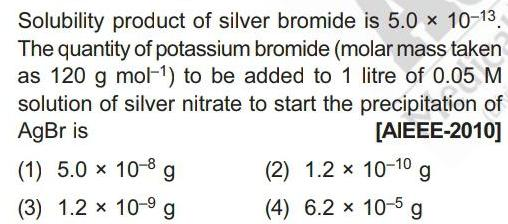Physical Chemistry
Equilibrium
Solubility product of silver bromide is 5 0 x 10 13 The quantity of potassium bromide molar mass taken as 120 g mol to be added to 1 litre of 0 05 M solution of silver nitrate to start the precipitation of AgBr is AIEEE 2010 1 5 0 10 8 g 3 1 2 x 10 9 g 2 1 2 x 10 1 g 4 6 2 x 10 5 g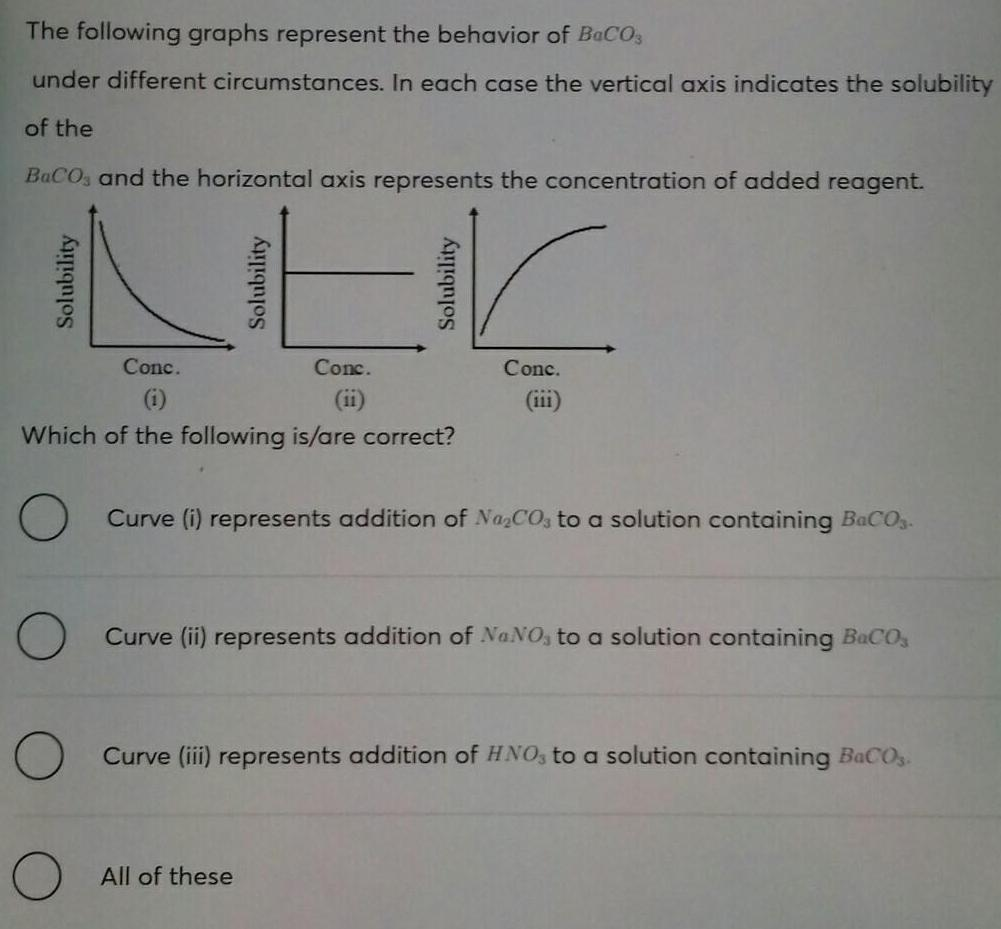Physical Chemistry
Equilibrium
The following graphs represent the behavior of BaCO3 under different circumstances In each case the vertical axis indicates the solubility of the BaCO and the horizontal axis represents the concentration of added reagent Solubility O Solubility Conc 1 Which of the following is are correct Solubility Conc ii All of these Conc Curve i represents addition of Na CO3 to a solution containing BaCO3 Curve ii represents addition of NaNO3 to a solution containing B CO Curve iii represents addition of HNO3 to a solution containing BaCO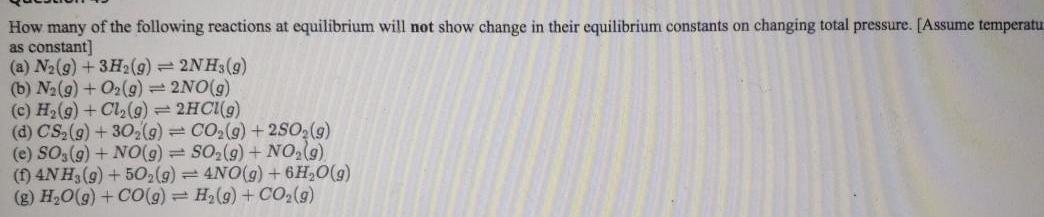Physical Chemistry
Equilibrium
How many of the following reactions at equilibrium will not show change in their equilibrium constants on changing total pressure Assume temperatu as constant a N g 3H g 2NH3 g b N g O2 g 2NO g c H g Cl g 2HCl g d CS g 30 g CO g 2SO g e SO3 g NO g SO g NO g f 4NH3 g 50 g 4NO g 6H O g g H O g CO g H g CO g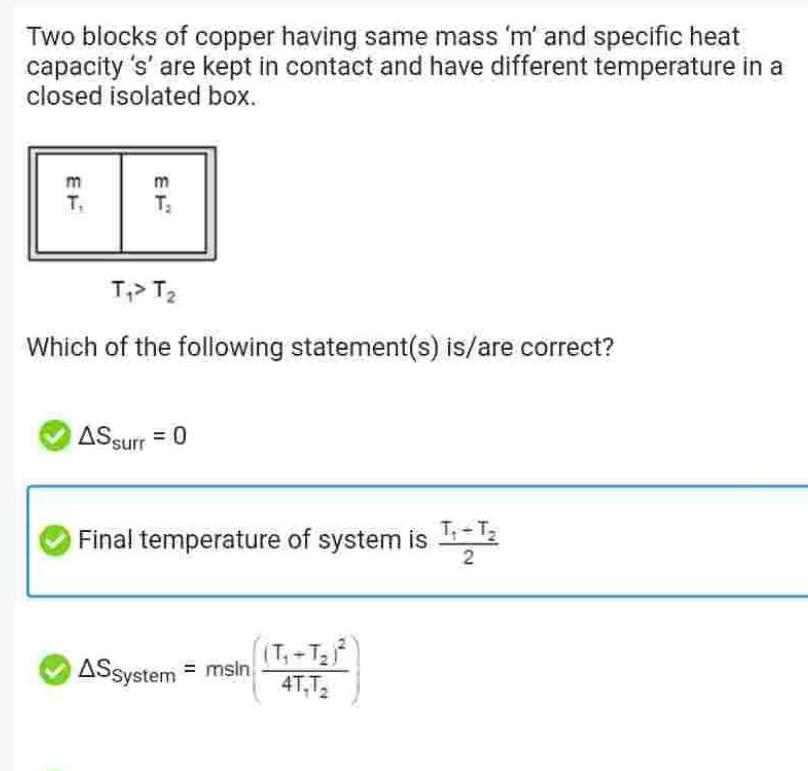Physical Chemistry
Equilibrium
Two blocks of copper having same mass m and specific heat capacity s are kept in contact and have different temperature in a closed isolated box m T T T T Which of the following statement s is are correct AS surr 0 Final temperature of system is T T ASSystem msin ATT2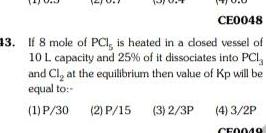Physical Chemistry
Equilibrium
CE0048 13 If 8 mole of PCI is heated in a closed vessel of 10 L capacity and 25 of it dissociates into PCI and Cl at the equilibrium then value of Kp will be equal to 1 P 30 2 P 15 3 2 3P 4 3 2P F0049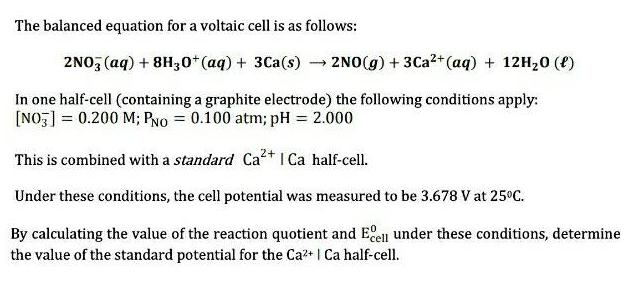Physical Chemistry
Equilibrium
The balanced equation for a voltaic cell is as follows 2NO3 aq 8H30 aq 3Ca s 2NO g 3Ca aq 12H 0 1 In one half cell containing a graphite electrode the following conditions apply NO3 0 200 M PNO 0 100 atm pH 2 000 This is combined with a standard Ca Ca half cell Under these conditions the cell potential was measured to be 3 678 V at 25 C By calculating the value of the reaction quotient and Ecell under these conditions determine the value of the standard potential for the Ca Ca half cell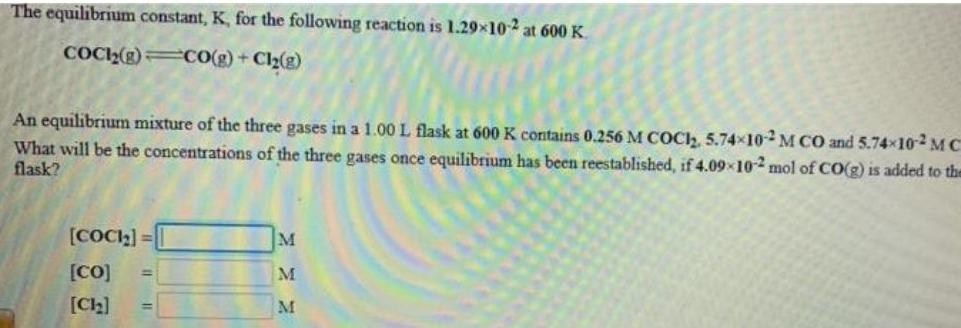Physical Chemistry
Equilibrium
The equilibrium constant K for the following reaction is 1 29 102 at 600 K COCI g CO g Cl g An equilibrium mixture of the three gases in a 1 00 L flask at 600 K contains 0 256 M COCI 5 74x10 2M CO and 5 74x102 MC What will be the concentrations of the three gases once equilibrium has been reestablished if 4 09 10 2 mol of CO g is added to the flask COCI CO Ch M M M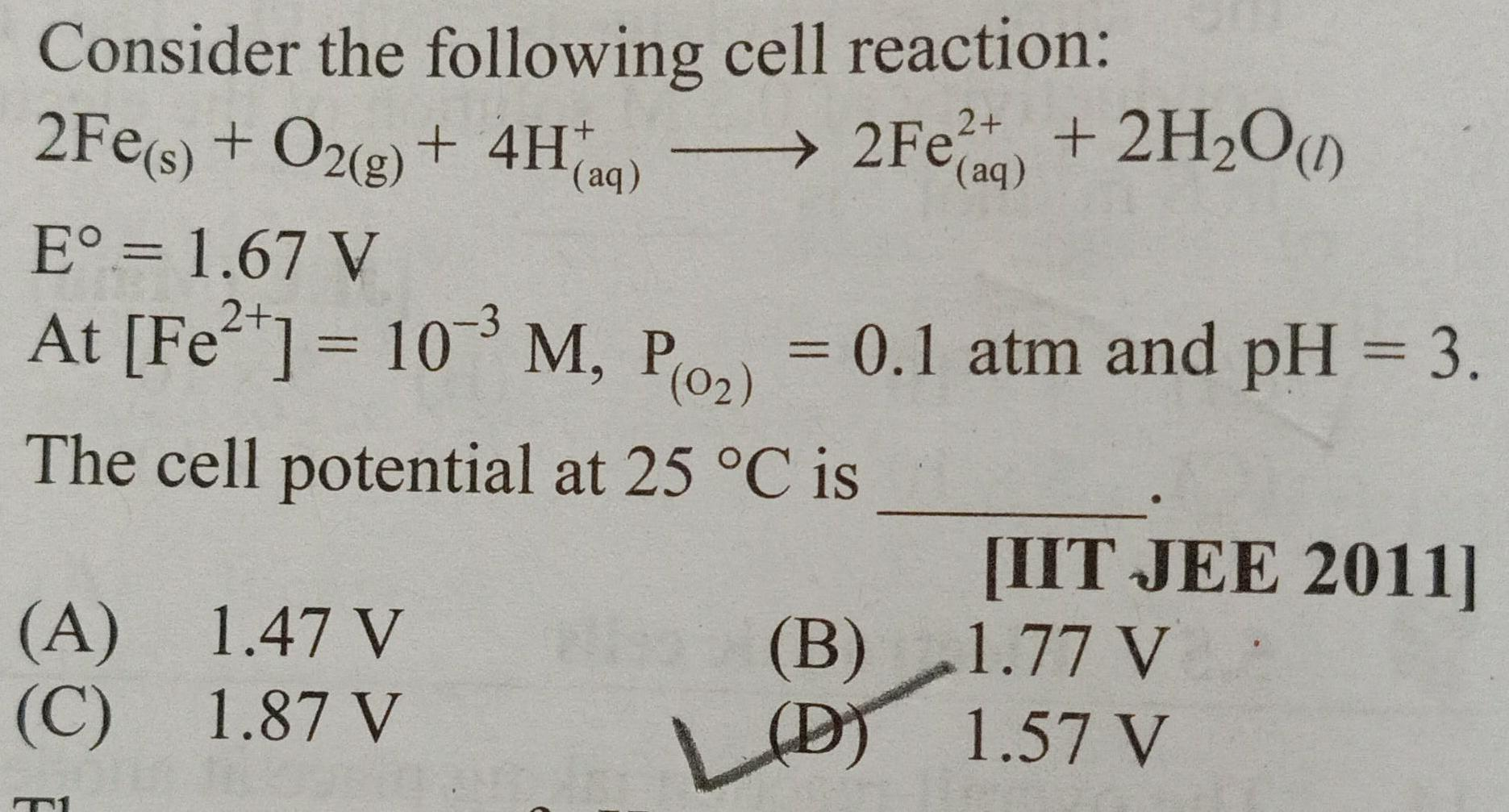Physical Chemistry
Equilibrium
Consider the following cell reaction 2Fe s O2 g 4H aq E 1 67 V 2 At Fe 10 M P 0 The cell potential at 25 C is A C 2Fe 2H O aq 1 47 V 1 87 V 0 1 atm and pH 3 B B LD IIT JEE 2011 1 77 V 1 57 V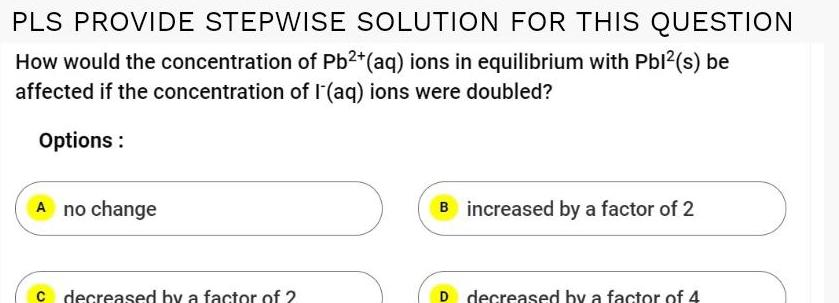Physical Chemistry
Equilibrium
PLS PROVIDE STEPWISE SOLUTION FOR THIS QUESTION How would the concentration of Pb2 aq ions in equilibrium with Pbl s be affected if the concentration of l aq ions were doubled Options A no change c decreased by a factor of 2 B increased by a factor of 2 D decreased by a factor of 4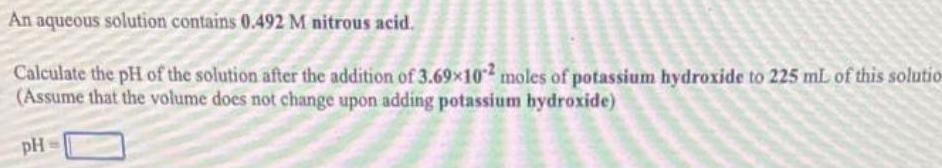Physical Chemistry
Equilibrium
An aqueous solution contains 0 492 M nitrous acid Calculate the pH of the solution after the addition of 3 69x102 moles of potassium hydroxide to 225 mL of this solutio Assume that the volume does not change upon adding potassium hydroxide pH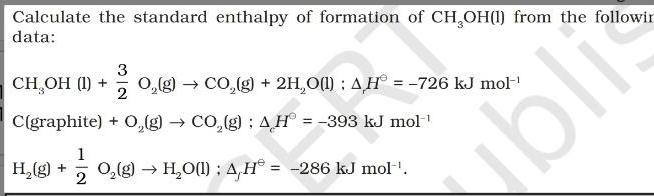Physical Chemistry
Equilibrium
Calculate the standard enthalpy of formation of CH OH 1 from the followin data 3 2 C graphite O g CO g AH 393 kJ mol CH OH 1 H g O g CO g 2H O 1 AH 726 kJ mol 1 O g H O l AH 286 kJ mol 2 bli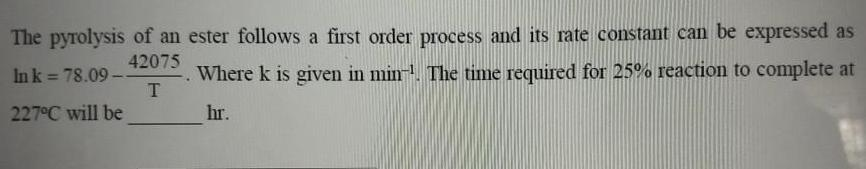Physical Chemistry
Equilibrium
The pyrolysis of an ester follows a first order process and its rate constant can be expressed as Where k is given in min The time required for 25 reaction to complete at 42075 In k 78 09 T 227 C will be hr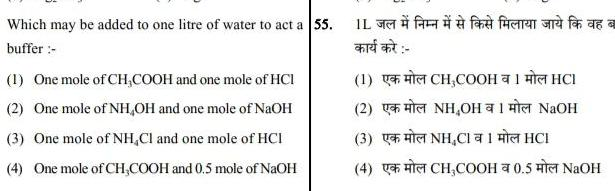Physical Chemistry
Equilibrium
Which may be added to one litre of water to act a 55 buffer 1 One mole of CH COOH and one mole of HCI 2 One mole of NH OH and one mole of NaOH 3 One mole of NH Cl and one mole of HCI 4 One mole of CH COOH and 0 5 mole of NaOH I fand fun and for as a anted and CH COOHHCI 1 2 3 U H CNH Ca 1 He HCI 4 NH NaH CH COOH 0 5 NaOH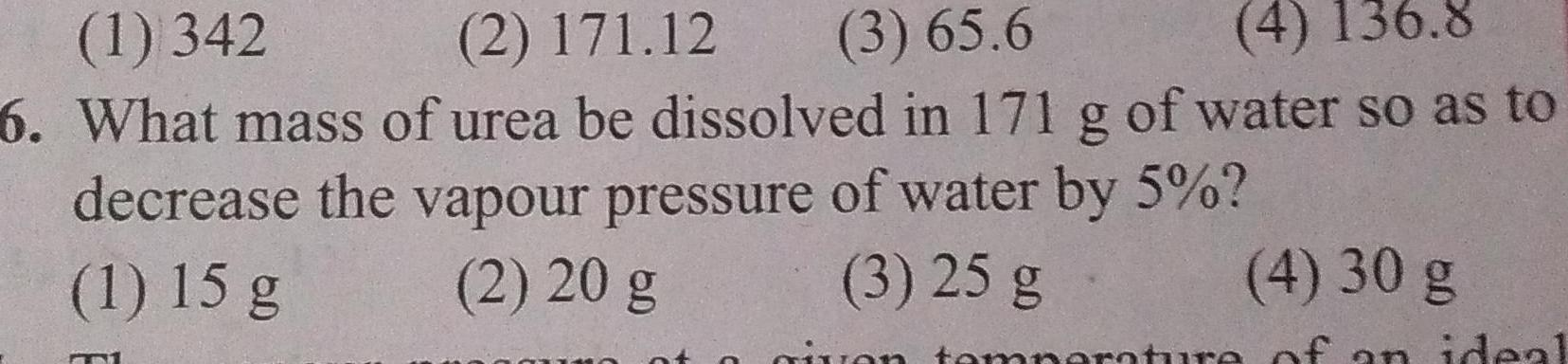Physical Chemistry
Equilibrium
1 342 2 171 12 3 65 6 4 136 8 6 What mass of urea be dissolved in 171 g of water so as to decrease the vapour pressure of water by 5 1 15 g 2 20 g 3 25 g vo 4 30 g mperature of ideal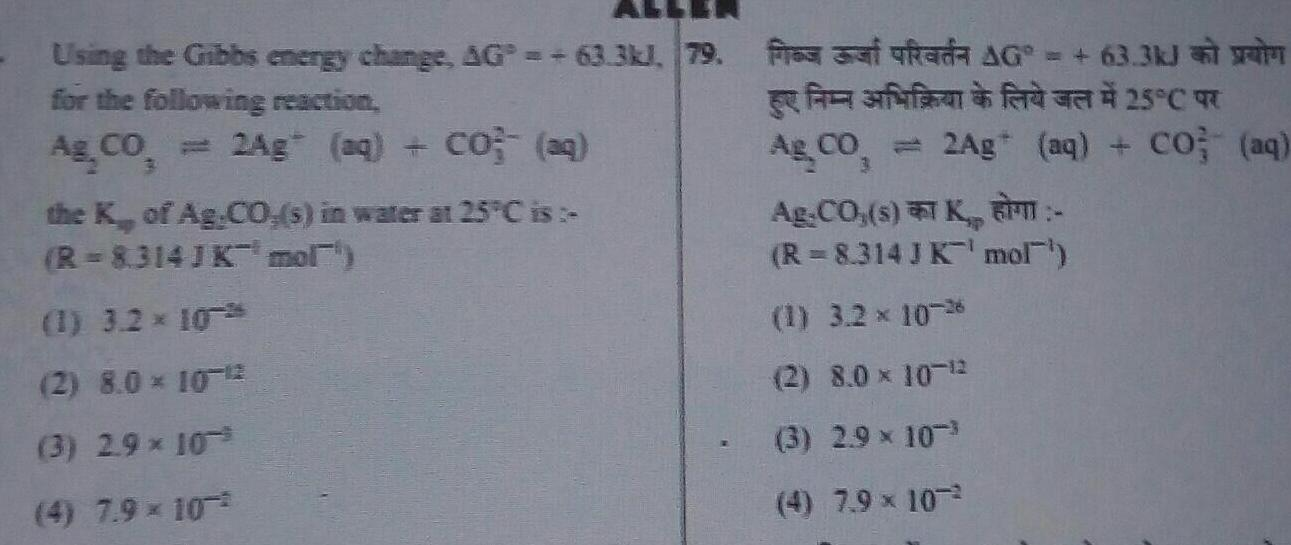Physical Chemistry
Equilibrium
Using the Gibbs energy change AG 63 3kJ 79 for the following reaction A CO 3 2Ag aq CO aq the K of Ag CO s in water at 25 C is R 8314J K mol 1 3 2 10 2 8 0 10 12 3 2 9 10 4 7 9 10 fres at Rada AG 63 3kJ ffff25 C Ag CO 2Ag aq CO aq 3 Ag CO s K R 8 314 J K mol 1 3 2 10 26 2 8 0 10 12 3 2 9 10 4 7 9 10 2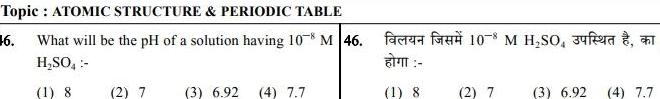Physical Chemistry
Equilibrium
Topic ATOMIC STRUCTURE PERIODIC TABLE 16 What will be the pH of a solution having 10 M 46 H SO4 1 8 2 7 3 6 92 4 7 7 1 8 10 M H SO 34 2 7 3 6 92 4 7 7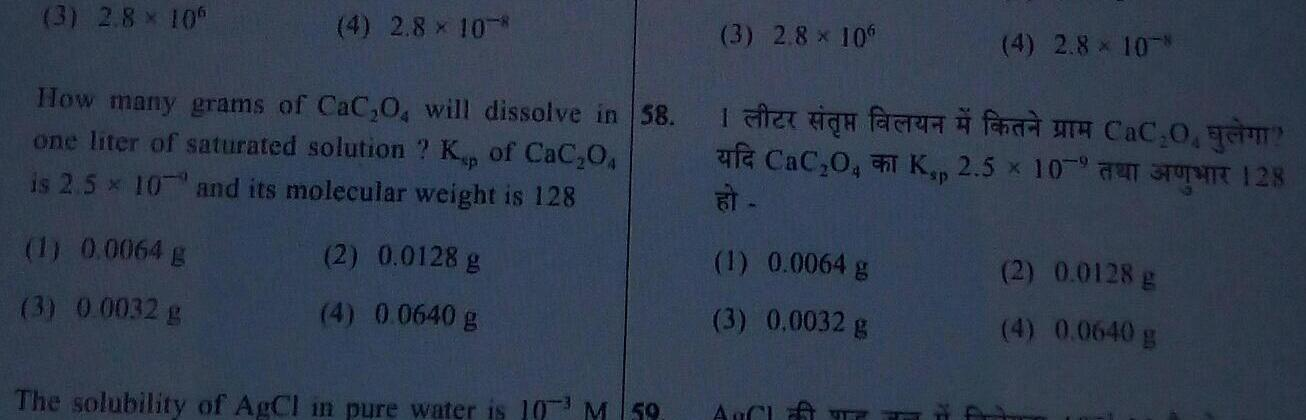Physical Chemistry
Equilibrium
3 2 8 106 4 2 8 10 How many grams of CaC 0 will dissolve in 58 one liter of saturated solution Kp of CaC 04 is 2 5x 10 and its molecular weight is 128 1 0 0064 g 3 0 0032 g 2 0 0128 g 4 0 0640 g The solubility of AgCl in pure water is 103 M 59 3 2 8 x 10 In fac a 0 yam af CaC O K 2 50 128 at 1 0 0064 g 3 0 0032 g 4 2 8 10 AUCH 2 0 0128 g 4 0 0640 g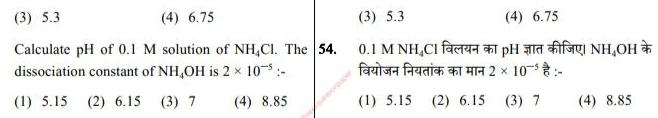Physical Chemistry
Equilibrium
3 5 3 4 6 75 Calculate pH of 0 1 M solution of NH CI The 54 dissociation constant of NH OH is 2 10 1 5 15 2 6 15 3 7 4 8 85 3 5 3 4 6 75 0 1 M NH CIH OH farise ferdian a 10 1 5 15 2 6 15 3 7 4 8 85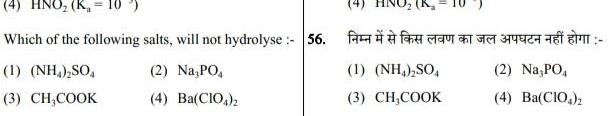Physical Chemistry
Equilibrium
4 HNO K 10 Which of the following salts will not hydrolyse 56 2 Na PO 4 Ba CIO4 2 1 NH4 SO4 3 CH COOK 1 NH4 SO4 3 CH COOK 2 Na PO 4 Ba CIO4 2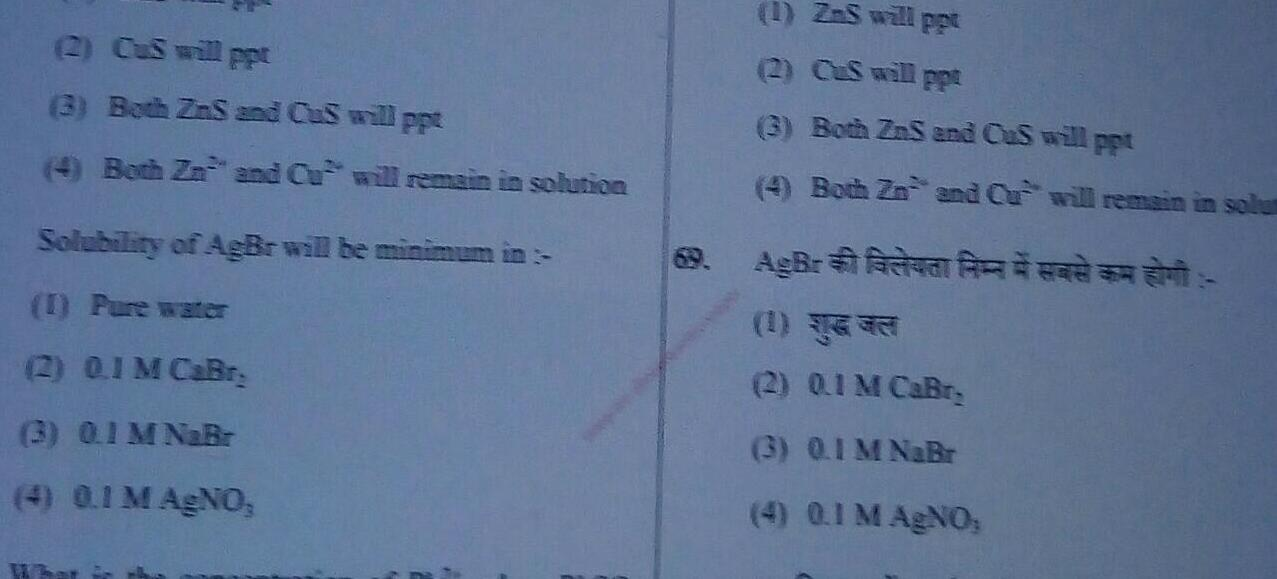Physical Chemistry
Equilibrium
2 CuS will ppt 3 Both ZnS and CuS will ppt 4 Both Zn and Cu will remain in solution Solubility of AgBr will be minimum in 1 Pure water 2 0 1 M CaBr 3 0 1 M NaBr 4 0 1 M AgNO 1 ZnS will ppt 2 CuS will ppt 3 Both ZnS and CuS will ppt 4 Both Zn and Cu will remain in sol AgB va 2 0 1 M CaBr 3 0 1 M NaBr 4 0 1 M AgNO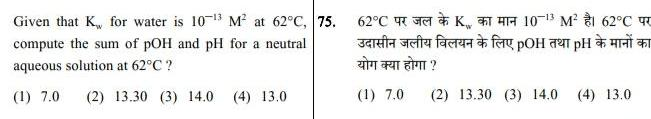Physical Chemistry
Equilibrium
Given that K for water is 10 3 M at 62 C 75 compute the sum of pOH and pH for a neutral aqueous solution at 62 C 1 7 0 2 13 30 3 14 0 4 13 0 62 C Ja fa fac H H K10 3 M 1 62 C T 1 7 0 2 13 30 3 14 0 4 13 0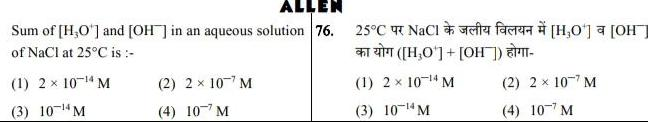Physical Chemistry
Equilibrium
ALLEN Sum of H O and OH in an aqueous solution 76 of NaCl at 25 C is 1 2 x 10 4 M 3 10 4 M 2 2 107 M 4 10 M 25 C NaCl H O OH 1 2 10 4 M 3 10 4 M 0 H 2 2 107 M 4 107 M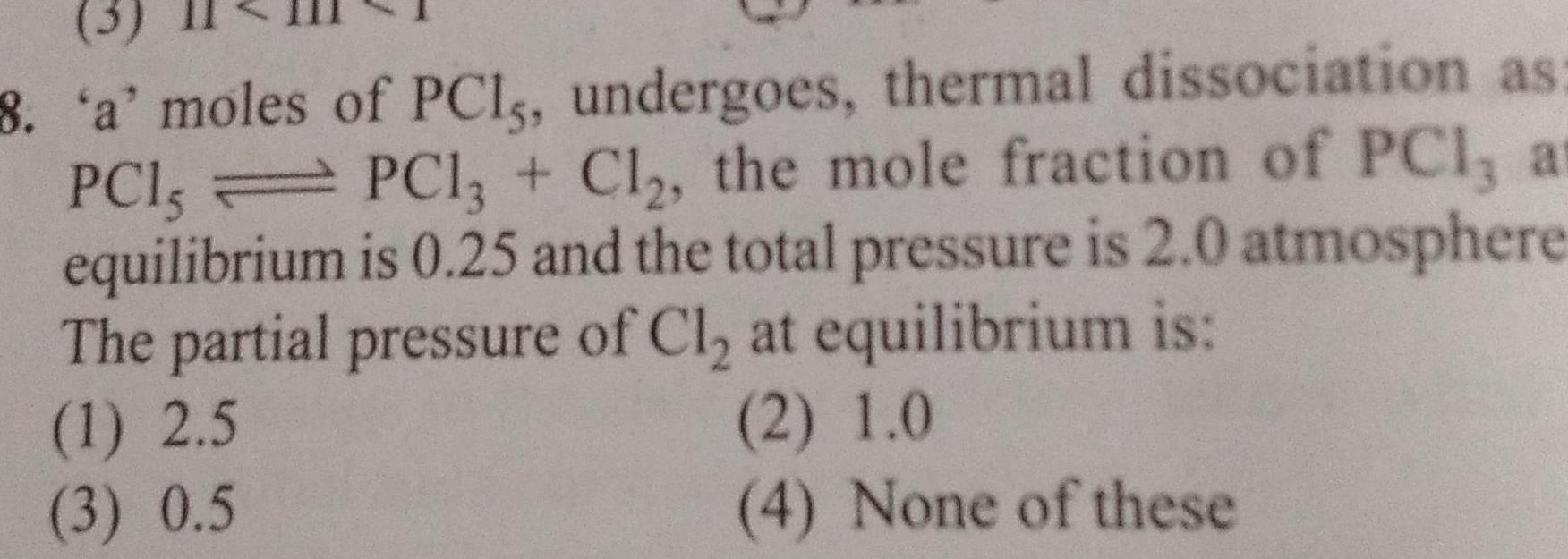Physical Chemistry
Equilibrium
8 a moles of PCI undergoes thermal dissociation as PC13 Cl the mole fraction of PCI at is 0 25 and the total pressure is 2 0 atmosphere The partial pressure of Cl at equilibrium is 1 2 5 PC1 equilibrium 2 1 0 3 0 5 4 None of these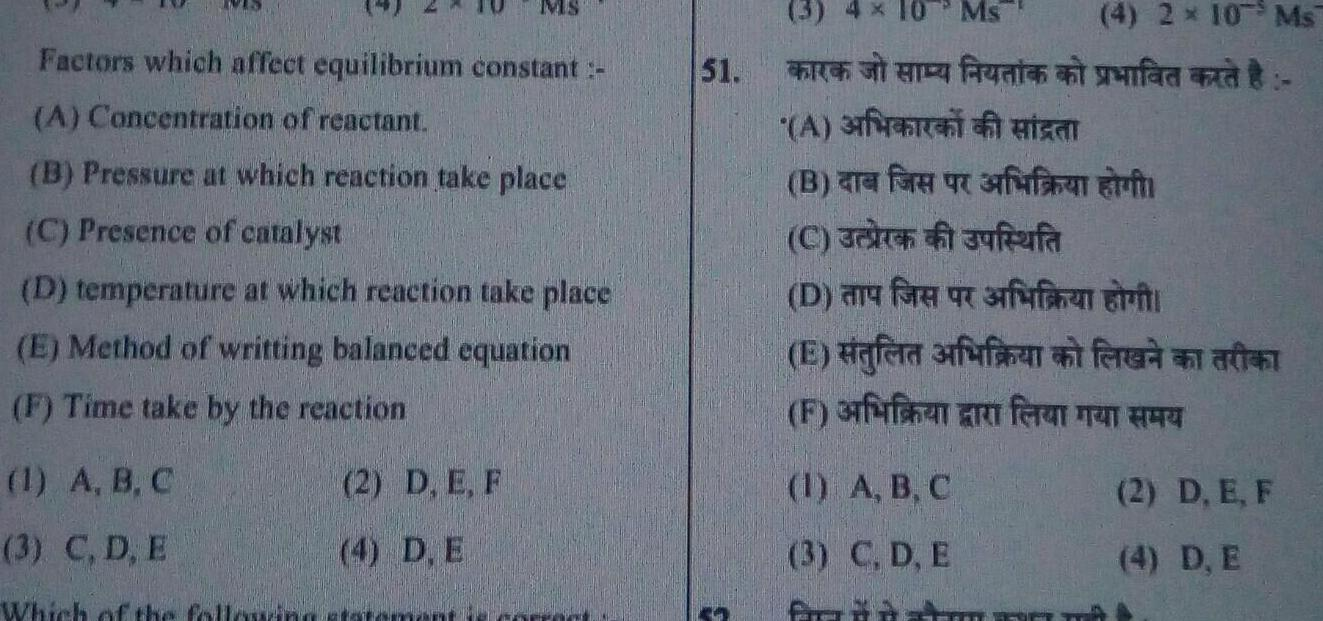Physical Chemistry
Equilibrium
Factors which affect equilibrium constant A Concentration of reactant B Pressure at which reaction take place C Presence of catalyst D temperature at which reaction take place E Method of writting balanced equation F Time take by the reaction 1 A B C 3 C D E Which of the following 2 D E F 4 D E 51 3 4 10 Ms funt A B C 31 Juftfa D and for fun 1 A B C 3 C D E fra 4 2 10 Ms ft risal forffenn E fera fuffra F erfurfhen a fer 2 D E F 4 D E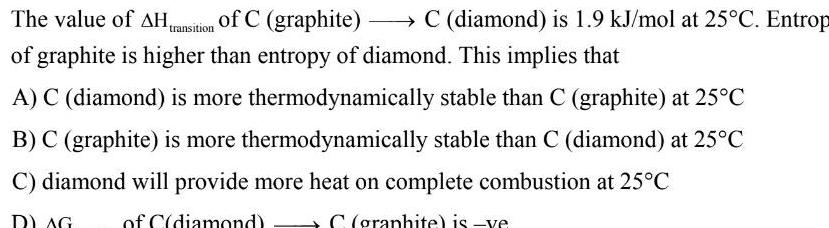Physical Chemistry
Equilibrium
The value of AH transition of C graphite C diamond is 1 9 kJ mol at 25 C Entrop of graphite is higher than entropy of diamond This implies that A C diamond is more thermodynamically stable than C graphite at 25 C B C graphite is more thermodynamically stable than C diamond at 25 C C diamond will provide more heat on complete combustion at 25 C DAG of C diamond C graphite is ve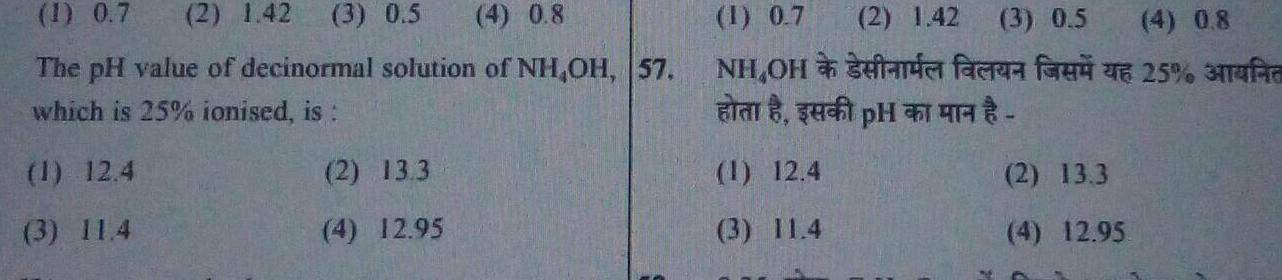Physical Chemistry
Equilibrium
1 0 7 2 1 42 3 0 5 4 0 8 The pH value of decinormal solution of NH OH 57 which is 25 ionised is 1 12 4 3 11 4 2 13 3 4 12 95 1 0 7 NH OH 1 12 4 3 11 4 2 1 42 3 0 5 ma faca fa 2 13 3 4 12 95 4 0 8 25 3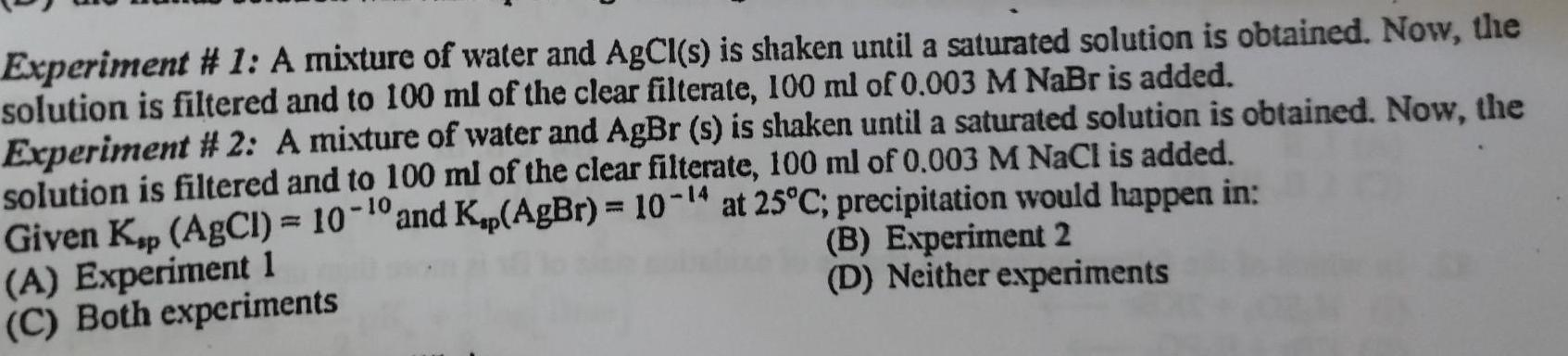Physical Chemistry
Equilibrium
Experiment 1 A mixture of water and AgCl s is shaken until a saturated solution is obtained Now the solution is filtered and to 100 ml of the clear filterate 100 ml of 0 003 M NaBr is added Experiment 2 A mixture of water and AgBr s is shaken until a saturated solution is obtained Now the solution is filtered and to 100 ml of the clear filterate 100 ml of 0 003 M NaCl is added Given Kp AgCl 10 10 and Kap AgBr 10 4 at 25 C precipitation would happen in B Experiment 2 D Neither experiments A Experiment 1 C Both experiments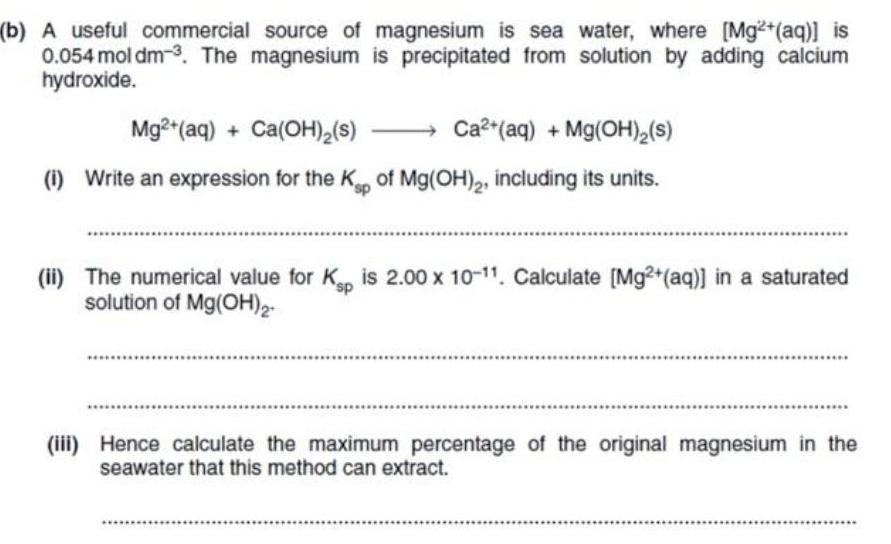Physical Chemistry
Equilibrium
b A useful commercial source of magnesium is sea water where Mg aq is 0 054 mol dm 3 The magnesium is precipitated from solution by adding calcium hydroxide Mg2 aq Ca OH s Ca aq Mg OH s i Write an expression for the Ksp of Mg OH 2 including its units ii The numerical value for Kp is 2 00 x 10 11 Calculate Mg aq in a saturated solution of Mg OH 2 iii Hence calculate the maximum percentage of the original magnesium in the seawater that this method can extract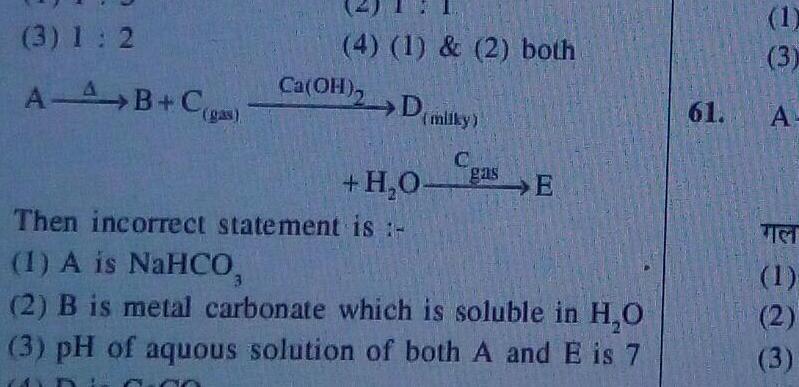Physical Chemistry
Equilibrium
3 1 2 AAB C gas 4 1 2 both Ca OH milky H O gas E Then incorrect statement is 1 A is NaHCO 2 B is metal carbonate which is soluble in H O 3 pH of aquous solution of both A and E is 7 61 3 A TIET 1 2 3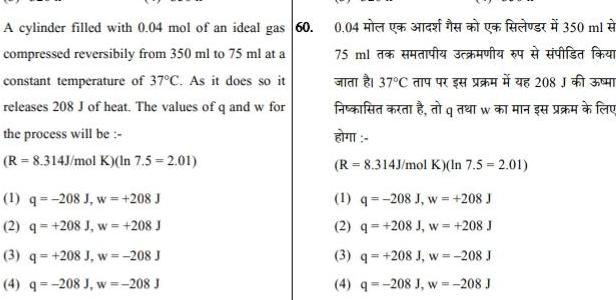Physical Chemistry
Equilibrium
A cylinder filled with 0 04 mol of an ideal gas 60 compressed reversibily from 350 ml to 75 ml at a constant temperature of 37 C As it does so it releases 208 J of heat The values of q and w for the process will be R 8 314J mol K In 7 5 2 01 1 q 208 J w 208 J 2 q 208 J w 208 J 3 q 208 J w 208 J 4 q 208 J w 208 J 0 04 3 350 ml 75 ml for ff at f 9 EFT R 8 314J mol K In 7 5 2 01 20 J 1 q 208 J w 208 J 2 q 208 J w 208 J 3 q 208 J w 208 J 4 q 208 J w 208 J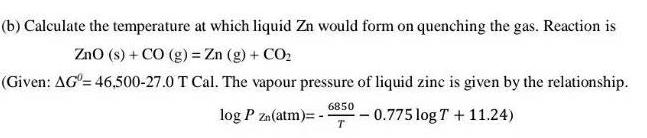Physical Chemistry
Equilibrium
b Calculate the temperature at which liquid Zn would form on quenching the gas Reaction is ZnO s CO g Zn g CO Given AG 46 500 27 0 T Cal The vapour pressure of liquid zinc is given by the relationship log P Zn atm 0 775 log T 11 24 6850 T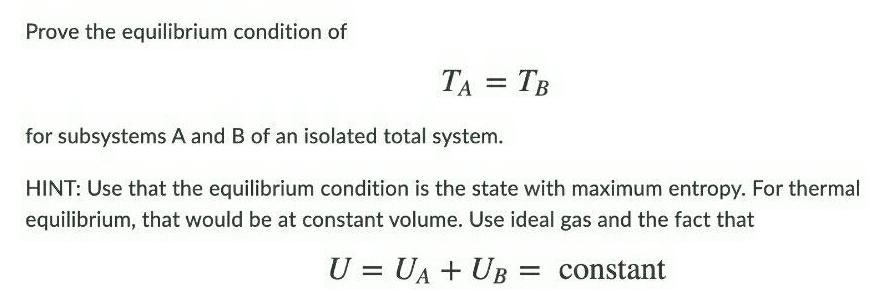Physical Chemistry
Equilibrium
Prove the equilibrium condition of TA TB for subsystems A and B of an isolated total system HINT Use that the equilibrium condition is the state with maximum entropy For thermal equilibrium that would be at constant volume Use ideal gas and the fact that U UA UB constant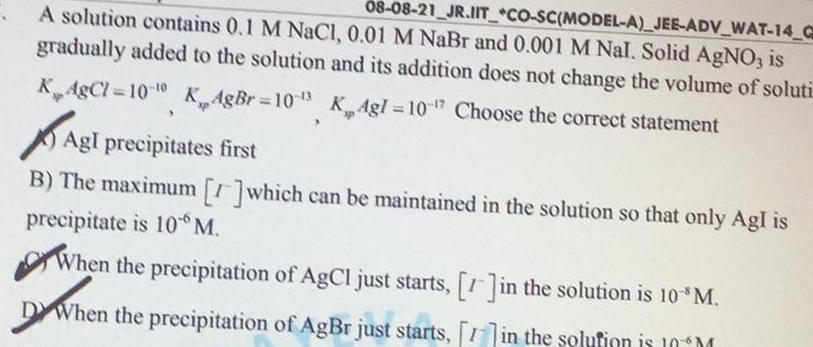Physical Chemistry
Equilibrium
A solution contains 0 1 M NaCl 0 01 M NaBr and 0 001 M Nal Solid AgNO3 is 08 08 21 JR IIT CO SC MODEL A JEE ADV WAT 14 C gradually added to the solution and its addition does not change the volume of soluti KAgCl 100 KAgBr 103 K Agl 10 17 Choose the correct statement 3 Agl precipitates first B The maximum which can be maintained in the solution so that only AgI is precipitate is 10 M When the precipitation of AgCl just starts 1 in the solution is 10 M DW When the precipitation of AgBr just starts 1 in the solution is 10 M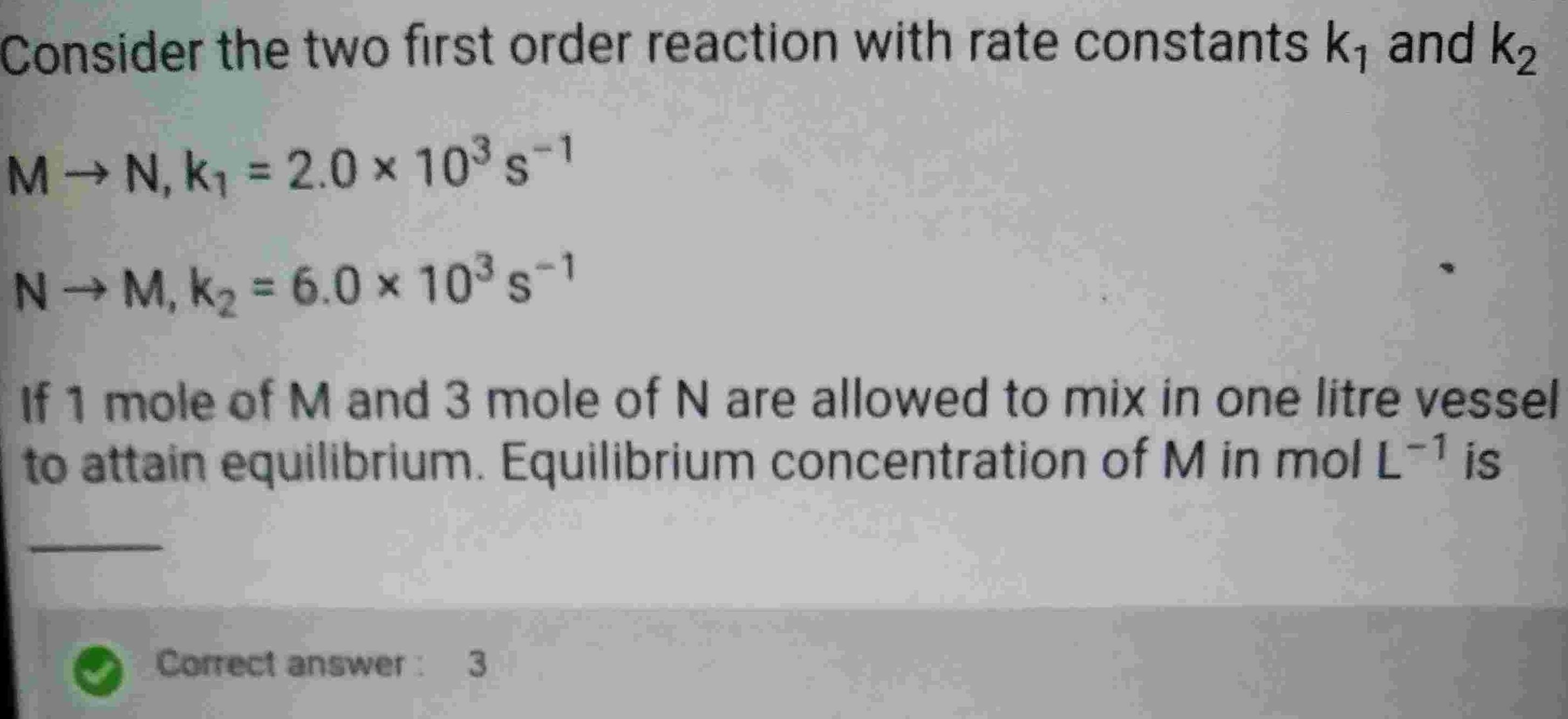Physical Chemistry
Equilibrium
Consider the two first order reaction with rate constants k and k M N K 2 0 10 s 1 N M K 6 0 10 s 1 If 1 mole of M and 3 mole of N are allowed to mix in one litre vessel to attain equilibrium Equilibrium concentration of M in mol L 1 is Correct answer 3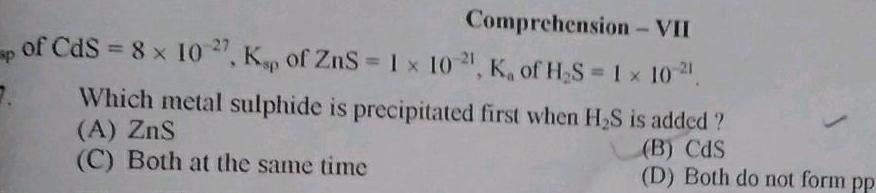Physical Chemistry
Equilibrium
Comprehension VII of CdS 8 x 102 Kp of ZnS 1 x 1021 K of H S 1 x 10 Which metal sulphide is precipitated first when H S is added A ZnS C Both at the same time B CdS D Both do not form pp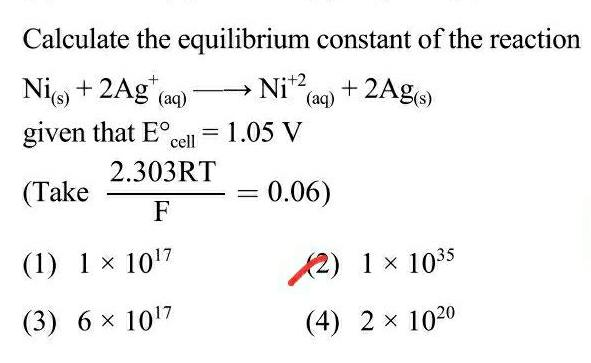Physical Chemistry
Equilibrium
Calculate the equilibrium Ni s 2Ag aq given that E Take 2 303RT F 1 1 10 7 3 6 1017 constant of the reaction 1 05 V cell Ni 2 2Ag s aq 0 06 2 1 1035 4 2 1020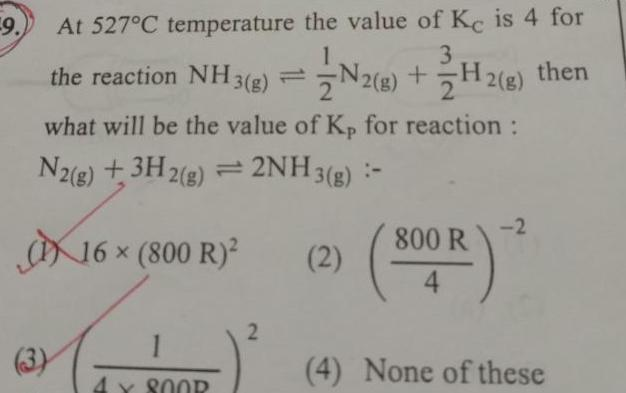Physical Chemistry
Equilibrium
9 At 527 C temperature the value of Ke is 4 for the reaction NH3 g what will be the value of Kp for reaction N2 g 3H2 g 2NH3 g 3 16 800 R X SOOR 2 2 g H2 g then 2 800 R 4 2 4 None of these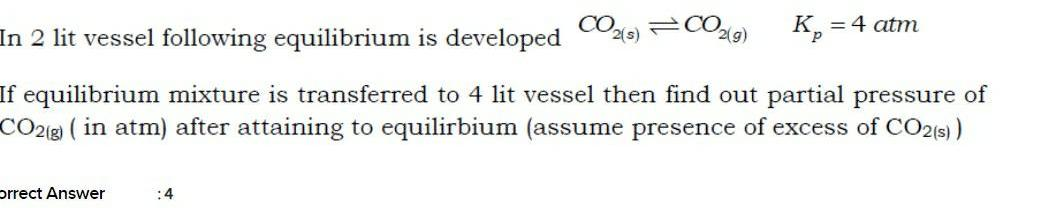Physical Chemistry
Equilibrium
In 2 lit vessel following equilibrium is developed CO2 s CO2 g If equilibrium mixture is transferred to 4 lit vessel then find out partial pressure of CO2 g in atm after attaining to equilirbium assume presence of excess of CO2 s Orrect Answer 4 atm 4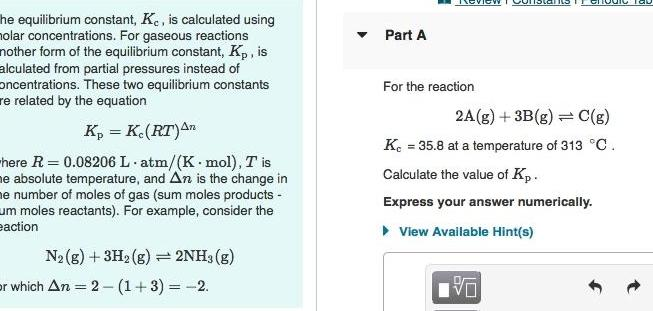Physical Chemistry
Equilibrium
he equilibrium constant Ke is calculated using holar concentrations For gaseous reactions nother form of the equilibrium constant Kp is alculated from partial pressures instead of oncentrations These two equilibrium constants re related by the equation Kp K RT An here R 0 08206 L atm K mol T is he absolute temperature and An is the change in me number of moles of gas sum moles products um moles reactants For example consider the eaction N g 3H2 g 2NH3 g or which An 2 1 3 2 Part A For the reaction 2A g 3B g C g K 35 8 at a temperature of 313 C Calculate the value of Kp Express your answer numerically View Available Hint s Die Tau VO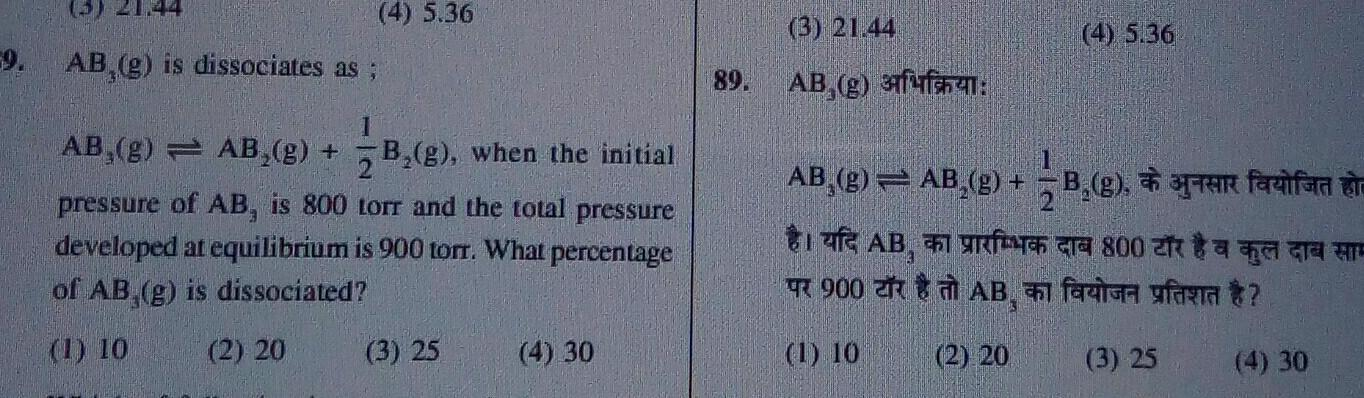Physical Chemistry
Equilibrium
3 21 44 59 AB g is dissociates as 4 5 36 1 AB g AB g B g when the initial pressure of AB is 800 torr and the total pressure developed at equilibrium is 900 torr What percentage of AB g is dissociated 1 10 2 20 3 25 4 30 89 3 21 44 AB g 3 AB g AB g B g aptar facifar dr AB80A TA TE AB faci fara 900 1 10 4 5 36 2 20 3 25 4 30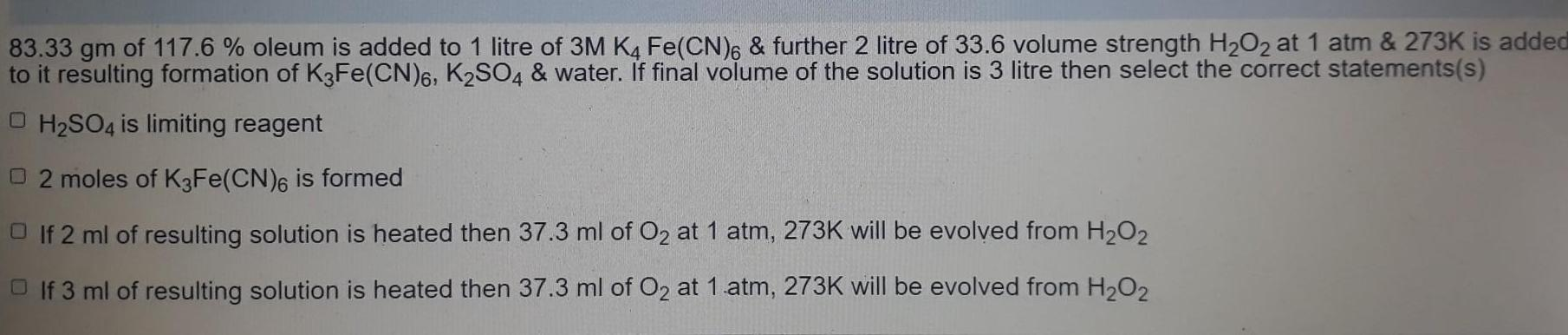Physical Chemistry
Equilibrium
83 33 gm of 117 6 oleum is added to 1 litre of 3M K4 Fe CN 6 further 2 litre of 33 6 volume strength H O2 at 1 atm 273K is added to it resulting formation of K3Fe CN 6 K2SO4 water If final volume of the solution is 3 litre then select the correct statements s O H SO4 is limiting reagent 2 moles of K3Fe CN 6 is formed If 2 ml of resulting solution is heated then 37 3 ml of O at 1 atm 273K will be evolved from H O If 3 ml of resulting solution is heated then 37 3 ml of O at 1 atm 273K will be evolved from H O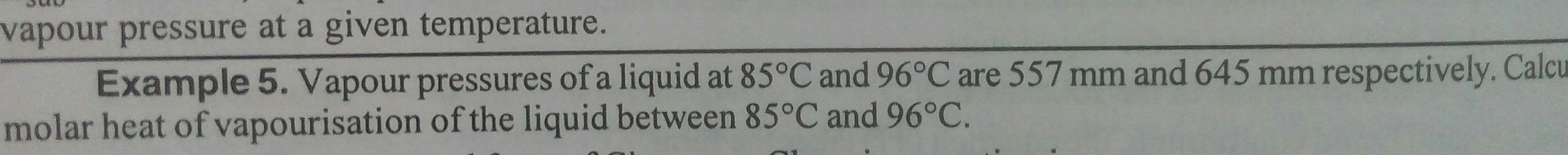Physical Chemistry
Equilibrium
vapour pressure at a given temperature Example 5 Vapour pressures of a liquid at 85 C and 96 C are 557 mm and 645 mm respectively Calcu molar heat of vapourisation of the liquid between 85 C and 96 C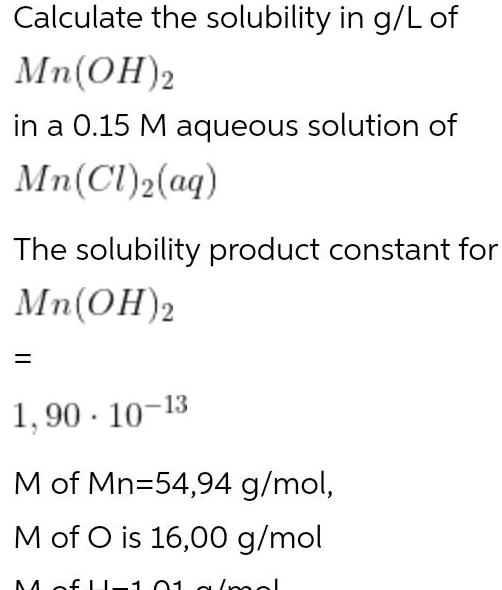Physical Chemistry
Equilibrium
Calculate the solubility in g L of Mn OH 2 in a 0 15 M aqueous solution of Mn Cl 2 aq The solubility product constant for Mn OH 2 1 90 10 13 M of Mn 54 94 g mol M of O is 16 00 g mol